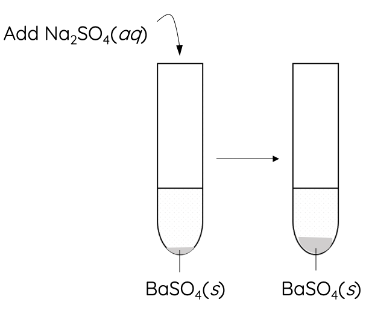# Common Ion Effect

This topic is part of HSC Chemistry course under the section Solution Equilibria.

### HSC Chemistry Syllabus

• Conduct an investigation to determine solubility rules, and predict and analyse the composition of substances when two ionic solutions are mixed, for example:

potassium chloride and silver nitrate

– potassium iodide and lead nitrate

– sodium sulfate and barium nitrate (ACSCH065)

• Predict the formation of a precipitate given the standard reference values for Ksp

### What is the common ion effect?

This video will explore the common ion effect which states that the addition of an ionic compound containing ions present in the equilibrium system, will have the same result as changing the concentration of that chemical species.

### Common Ion Effect

• According to Le Châtelier’s principle, changing the concentration of chemical species of an equilibrium system will disturb and move the position of the equilibrium. Addition of an ionic compound that contains an ion present in the equilibrium system will achieve the same result. This is known as the common ion effect.

Example 1 – Barium sulfate solution

• Addition of sodium sulfate to a saturated solution of barium sulfate increases the amount of barium sulfate precipitate.• This is because, according to Le Chatelier’s principle, an increase in [SO42–] causes the equilibrium position to shift to the left in order to reduce [SO42–]. As a result, [Ba2+] decreases and the amount of BaSO4(s) increases.• Addition of sodium sulfate reduces the solubility of barium sulfate
• Concentrations of ions change in a specific way such that the solubility product constant, Ksp, remains constant. This means the final [SO42–] > initial [SO42–] and final [Ba2+] < initial [Ba2+]

Example 2 – Lead(II) chromate solution

• Consider a saturated solution of lead(II) chromate:

$$PbCrO_{4(s)}\rightleftharpoons {\rm Pb}_{(aq)}^{2+}+CrO_{4(aq)}^{2-}\ \ \ \ K_{sp}=\left[{\rm Pb}_{\left(aq\right)}^{2+}\right]\left[CrO_{4\left(aq\right)}^{2-}\right]=2.3\times{10}^{-13}$$

• Suppose Na2CrO4, a soluble salt is added to the saturated PbCrO4 The concentration of the common ion, CrO42-, increases, and some of it combines with Pb2+ ion to form more solid PbCrO4 (figure below). In effect, the addition of Na2CrO4 will shift the equilibrium to the left.• As a result of the addition, [Pb2+] is reduced. And, since [Pb2+] defines the solubility of PbCrO4, in effect, the solubility of PbCrO4 has decreased.
• The same result would occur if Na2CrO4 solution was the solvent; that is, PbCrO4 is more soluble in water than in aqueous Na2CrO4.

In general:

• Addition of a common ion reduces the solubility of the ionic compound.
• Removal of a common ion increases the solubility of the ionic compound.
• Adding or removal common ions does not change the value of the solubility product, Ksp.

## Practice Questions

Question 1

Given the Ksp for calcium sulfate is 9.1 \times 10^{–6}, determine whether a solution which contains calcium ions at a concentration of 1.00 mol L^{–1} and sulfate ions at a concentration of 2.56 mol L^{–1} is saturated with calcium sulfate.

Question 2

Given that Ksp BaSO4 = [Ba2+] [SO42-] = 1.1 \times 10^{–10}, determine the solubility of barium sulfate in a solution of sodium sulfate of a concentration 1.00 mol L^{–1}

Previous Section: Calculating Solubility Product Quotient Q

Next Section: Effect of Temperature on Solubility